# Types of Logaritms

Although a logarithm may be defined with any base, the logs most often encountered are the logarithm to the base 10 which is called the common logarithm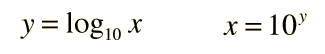and the logarithm to the base e which is called the natural logarithm.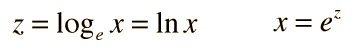Base changes can be accomplished. For a chosen base: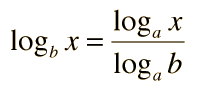Logarithms may be manipulated with the combination rules.

Index

 HyperPhysics*****HyperMath*****Logarithms R Nave
Go Back

# Logarithm Combinations

The logarithm to the base b of the variable x is defined as the power to which you would raise b to get x. It is written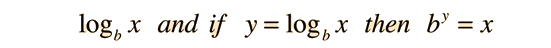.

The general combination properties of logarithms are: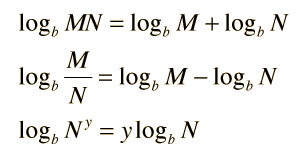Index

 HyperPhysics*****HyperMath*****Logarithms R Nave
Go Back

# Logarithm Base Change

If the logarithm to the base a is known, then the logarithm to the base b can be obtained by the base change relationship:This can be proved from the definition and combination rules for logarithms. Ifthen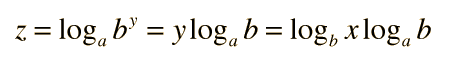and rearranging givesThe most common base changes are from the natural log to base 10 log or vice versa.

log10x = ln x/ln 10 = ln x/2.30258 = 0.4343 ln x
Index

Complex numbers

 HyperPhysics*****HyperMath*****Logarithms R Nave
Go Back

# What is a Logarithm?

The logarithm to the base b of the variable x is defined as the power to which you would raise b to get x. If the logarithm to the base b of x is equal to y, then b raised to the y power will give you the value x. It it written.

The general combination properties of logarithms are:Index

 HyperPhysics*****HyperMath*****Logarithms R Nave
Go Back